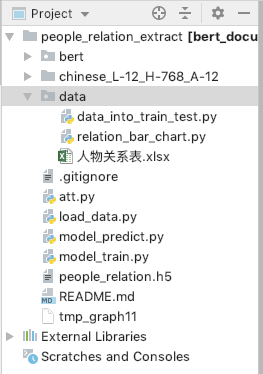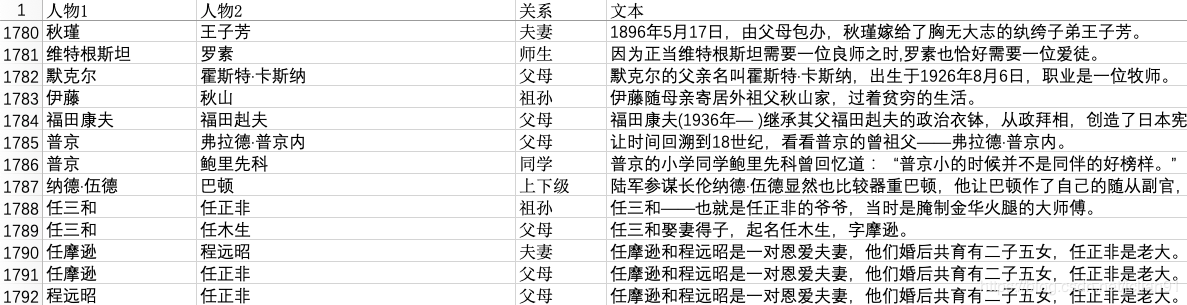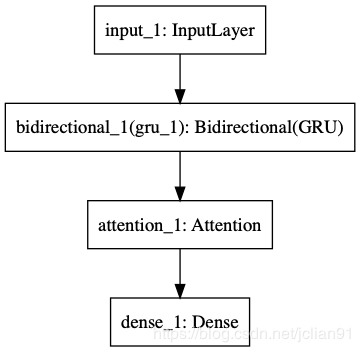# NLP（二十一）人物关系抽取的一次实战

2019/04/10 10:10

去年，笔者写过一篇文章利用关系抽取构建知识图谱的一次尝试，试图用现在的深度学习办法去做开放领域的关系抽取，但是遗憾的是，目前在开放领域的关系抽取，还没有成熟的解决方案和模型。当时的文章仅作为笔者的一次尝试，在实际使用过程中，效果有限。   本文将讲述如何利用深度学习模型来进行人物关系抽取。人物关系抽取可以理解为是关系抽取，这是我们构建知识图谱的重要一步。本文人物关系抽取的主要思想是关系抽取的pipeline（管道）模式，因为人名可以使用现成的NER模型提取，因此本文仅解决从文章中抽取出人名后，如何进行人物关系抽取。   本文采用的深度学习模型是文本分类模型，结合BERT预训练模型，取得了较为不错的效果。   本项目已经开源，Github地址为：https://github.com/percent4/people_relation_extract 。   本项目的项目结构图如下：### 数据集介绍

在进行这方面的尝试之前，我们还不得不面对这样一个难题，那就是中文人物关系抽取语料的缺失。数据是模型的前提，没有数据，一切模型无从谈起。因此，笔者不得不花费大量的时间收集数据。   笔者利用大量自己业余的时间，收集了大约2900条人物关系样本，整理成Excel（文件名称为人物关系表.xlsx），其中几行如下：人物关系一共有14类，分别为unknown,夫妻,父母,兄弟姐妹,上下级,师生,好友,同学,合作,同人,情侣,祖孙,同门,亲戚，其中unknown类别表示该人物关系不在其余的13类中（人物之间没有关系或者为其他关系），同人关系指的是两个人物其实是同一个人，比如下面的例子：

邵逸夫(1907年10月4日—2014年1月7日)，原名邵仁楞，生于浙江省宁波市镇海镇，祖籍浙江宁波。


# -*- coding: utf-8 -*-
# 绘制人物关系频数统计条形图
import pandas as pd
import matplotlib.pyplot as plt

# 读取EXCEL数据
label_list = list(df['关系'].value_counts().index)
num_list= df['关系'].value_counts().tolist()

# Mac系统设置中文字体支持
plt.rcParams["font.family"] = 'Arial Unicode MS'

# 利用Matplotlib绘制条形图
x = range(len(num_list))
rects = plt.bar(left=x, height=num_list, width=0.6, color='blue', label="频数")
plt.ylim(0, 500) # y轴范围
plt.ylabel("数量")
plt.xticks([index + 0.1 for index in x], label_list)
plt.xticks(rotation=45) # x轴的标签旋转45度
plt.xlabel("人物关系")
plt.title("人物关系频数统计")
plt.legend()

# 条形图的文字说明
for rect in rects:
height = rect.get_height()
plt.text(rect.get_x() + rect.get_width() / 2, height+1, str(height), ha="center", va="bottom")

plt.show()


### 数据预处理

收集好数据后，我们需要对数据进行预处理，预处理主要分两步，一步是将人物关系和原文本整合在一起，第二步简单，将数据集划分为训练集和测试集，比例为8:2。   我们对第一步进行详细说明，将人物关系和原文本整合在一起。一般我们给定原文本和该文本中的两个人物，比如：

邵逸夫(1907年10月4日—2014年1月7日)，原名邵仁楞，生于浙江省宁波市镇海镇，祖籍浙江宁波。


texts.append(text)

df['text'] = texts

train_df = df.sample(frac=0.8, random_state=1024)
test_df = df.drop(train_df.index)

with open('train.txt', 'w', encoding='utf-8') as f:
for text, rel in zip(train_df['text'].tolist(), train_df['rel'].tolist()):
f.write(str(rel)+' '+text+'\n')

with open('test.txt', 'w', encoding='utf-8') as g:
for text, rel in zip(test_df['text'].tolist(), test_df['rel'].tolist()):
g.write(str(rel)+' '+text+'\n')


{
"unknown": 0,
"夫妻": 1,
"父母": 2,
"兄弟姐妹": 3,
"上下级": 4,
"师生": 5,
"好友": 6,
"同学": 7,
"合作": 8,
"同人": 9,
"情侣": 10,
"祖孙": 11,
"同门": 12,
"亲戚": 13
}


4 方琳$李伟康$在生活中，###则把##看作小辈，常常替她解决难题。
3 佳子$久仁$12月，##和弟弟##参加了在东京举行的全国初中生演讲比赛。
2 钱慧安$钱禄新$###，生卒年不详，海上画家###之子。
0 吴继坤$邓新生$###还曾对媒体说：“我这个小小的投资商，经常得到###等领导的亲自关注和关照，我觉到受宠若惊。”
2 洪博培$乔恩·M·亨茨曼$###的父亲########是著名企业家、美国最大化学公司亨茨曼公司创始人。
10 夏乐$陈飞$两小无猜剧情简介:##和##是一对从小一起长大的青梅竹马。


### 模型训练

在模型训练前，为了将数据的格式更好地适应模型，需要再对trian.txt和test.txt进行处理。处理脚本为load_data.py，完整的Python代码如下：

# -*- coding: utf-8 -*-
import pandas as pd

# 读取txt文件
with open(file_path, 'r', encoding='utf-8') as f:
content = [_.strip() for _ in f.readlines()]

labels, texts = [], []
for line in content:
parts = line.split()
label, text = parts, ''.join(parts[1:])
labels.append(label)
texts.append(text)

return labels, texts

# 获取训练数据和测试数据，格式为pandas的DataFrame
def get_train_test_pd():
file_path = 'data/train.txt'
train_df = pd.DataFrame({'label': labels, 'text': texts})

file_path = 'data/test.txt'
test_df = pd.DataFrame({'label': labels, 'text': texts})

return train_df, test_df

if __name__ == '__main__':

train_df, test_df = get_train_test_pd()

train_df['text_len'] = train_df['text'].apply(lambda x: len(x))
print(train_df.describe())


本项目所采用的模型为：BERT + 双向GRU + Attention + FC，其中BERT用来提取文本的特征，关于这一部分的介绍，已经在文章NLP（二十）利用BERT实现文本二分类中给出；Attention为注意力机制层，FC为全连接层，模型的结构图如下（利用Keras导出）：模型训练的脚本为model_train.py，完整的Python代码如下：

# -*- coding: utf-8 -*-
# 模型训练

import numpy as np
from keras.utils import to_categorical
from keras.models import Model
from keras.layers import Input, Dense
from bert.extract_feature import BertVector

from att import Attention
from keras.layers import GRU, Bidirectional

# 读取文件并进行转换
train_df, test_df = get_train_test_pd()
bert_model = BertVector(pooling_strategy="NONE", max_seq_len=80)
print('begin encoding')
f = lambda text: bert_model.encode([text])["encodes"]

train_df['x'] = train_df['text'].apply(f)
test_df['x'] = test_df['text'].apply(f)
print('end encoding')

# 训练集和测试集
x_train = np.array([vec for vec in train_df['x']])
x_test = np.array([vec for vec in test_df['x']])
y_train = np.array([vec for vec in train_df['label']])
y_test = np.array([vec for vec in test_df['label']])
# print('x_train: ', x_train.shape)

# 将类型y值转化为ont-hot向量
num_classes = 14
y_train = to_categorical(y_train, num_classes)
y_test = to_categorical(y_test, num_classes)

# 模型结构：BERT + 双向GRU + Attention + FC
inputs = Input(shape=(80, 768,))
gru = Bidirectional(GRU(128, dropout=0.2, return_sequences=True))(inputs)
attention = Attention(32)(gru)
output = Dense(14, activation='softmax')(attention)
model = Model(inputs, output)

# 模型可视化
# from keras.utils import plot_model
# plot_model(model, to_file='model.png')

model.compile(loss='categorical_crossentropy',
metrics=['accuracy'])

# 模型训练以及评估
model.fit(x_train, y_train, batch_size=8, epochs=30)
model.save('people_relation.h5')
print(model.evaluate(x_test, y_test))


begin encoding
end encoding
Epoch 1/30
1433/1433 [==============================] - 15s 10ms/step - loss: 1.5558 - acc: 0.4962
**********(中间部分省略输出)**************
Epoch 30/30
1433/1433 [==============================] - 12s 8ms/step - loss: 0.0210 - acc: 0.9951
[1.1099, 0.7709]


### 模型预测

上述模型训练完后，利用保存好的模型文件，对新的数据进行预测。模型预测的脚本为model_predict.py，完整的Python代码如下：

# -*- coding: utf-8 -*-
# 模型预测

import json
import numpy as np
from bert.extract_feature import BertVector
from att import Attention

# 加载模型

# 示例语句及预处理
text = '赵金闪#罗玉兄#在这里，赵金闪和罗玉兄夫妇已经生活了大半辈子。他们夫妇都是哈密市伊州区林业和草原局的护林员，扎根东天山脚下，守护着这片绿。'
per1, per2, doc = text.split('#')
text = '\$'.join([per1, per2, doc.replace(per1, len(per1)*'#').replace(per2, len(per2)*'#')])
print(text)

# 利用BERT提取句子特征
bert_model = BertVector(pooling_strategy="NONE", max_seq_len=80)
vec = bert_model.encode([text])["encodes"]
x_train = np.array([vec])

# 模型预测并输出预测结果
predicted = model.predict(x_train)
y = np.argmax(predicted)

with open('data/rel_dict.json', 'r', encoding='utf-8') as f:

id_rel_dict = {v:k for k,v in rel_dict.items()}
print(id_rel_dict[y])


原文: 润生#润叶#不过，他对润生的姐姐润叶倒怀有一种亲切的感情。



### 总结

本文采用的深度学习模型是文本分类模型，结合BERT预训练模型，在小标注数据量下对人物关系抽取这个任务取得了还不错的效果。同时模型的识别准确率和使用范围还有待于提升，提升点笔者认为如下：

• 标注的数据量需要加大，现在的数据才2900条左右，如果数据量上去了，那么模型的准确率还有使用范围也会提升；
• 其他更多的模型有待于尝试；
• 在预测时，模型的预测时间较长，原因在于用BERT提取特征时耗时较长，可以考虑缩短模型预测的时间（比如使用ALBERT就能大大缩短预测时间）；
• 其他问题欢迎补充。

感谢大家阅读~

0
0 收藏

### 作者的其它热门文章0 评论
0 收藏
0